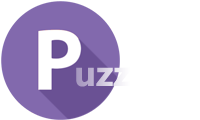# IQ Test Labs

Discover your intellectual strengths### Mathematics brain teasers

1. You begin reading a book 240 pages long. If you read half of the remaining book each day how long would it take you to finish the book?

You will never finish the book.

2. Add a single line to the equation in order to make it true.

105 + 2 + 5 = 350

Add a line to the second plus sign in order to make it into a four.

105 + 245 = 350

3. Find the product of the following series:

(x-a) (x-b) (x-c) ..... (x-z)

Zero. All factors are multiplied by the factor (x-x) which is zero.

4. What number when multiplied against itself will result in a number which includes the numbers (1 - 9) in forward order, and then descending in order from the 9? (i.e., to get the number: 12,345,678,987,654,321)

111,111,111 X 111,111,111 = 12,345,678,987,654,321

5. An old man said to a young man, "I have a daughter. She has as many brothers as she has sisters. Each one of her brothers has twice as many sisters as he has brothers. How many sons and daughters do I have?

3 sons and 4 daughters

6. A kite and surfboard cost \$1500 in total. The kite costs \$1,000 more than the surfboard. How much does the surfboard cost?

\$250